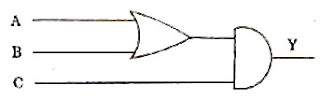## Tuesday, April 20, 2010

### AIPMT 2010 Multiple Choice Questions (MCQ) on Electronics

Questions on electronics at the level expected from you in most of the entrance examinations are generally simple. (You won't find questions in this section in IIT-JEE and AP Physics Exam question papers). Here are the five questions on electronics (including digital circuits) which appeared in the AIPMT 2010 question paper:

(1) A common emitter amplifier has a voltage gain of 50, an input impedance of 100 Ω and an output impedance of 200 Ω. The power gain of the amplifier is

(1) 50

(2) 500

(3) 1000

(4) 1250

In the case of common emitter amplifier, the voltage gain Av is given by

Av = βac(Ro/Ri) where βac is the a.c. current gain, Ro is the output resistance and Ri is the intput resistance. (Instead of Ro and Ri you may use Zo and Zi, the respective impedances). The negative sign just indicates the 180º phase shift of the output signal with respect to the input signal.

Therefore we have 50 = βac×200/100 from which βac = 25.

Power gain = current gain×voltage gain = 25×50 = 1250.

(2) Which one of the following bonds produces a solid that reflects light in the visible region and whose electrical conductivity decreases with temperature and has high melting point?

(1) Covalent bonding

(2) Metallic bonding

(3) van der Waal's bonding

(4) Ionic bonding

This is a very simple question and the answer is metallic bonding.

(3) The device that can act as a complete electronic circuit is

(1) Zener diode

(2) Junction diode

(3) Integrated circuit

(4) Junction transistor

This too is a very simple question and the answer is integrated circuit.

(4) Which one of the following statement is FALSE ?

(1) The resistance of intrinsic semiconductor decreases with increase of temperature

(2) Pure Si doped with trivalent impurities gives a p-type semiconductor

(3) Majority carriers in a n-type semiconductor are holes

(4) Minority carries in a p-type semiconductor are electrons

The FALSE statement is given in option (3).

(5) To get an output Y = 1 from the circuit shown below, the input must be---A B C---

(1) 1 0 0

(2) 0 1 0

(3) 0 0 1

(4) 1 0 1

The correct option is (4) since the circuit implements the operation Y = (A+B).C

You will find the same question and some other questions on digital circuits with solution here: Karnataka CET Questions (MCQ) on Digital Circuits